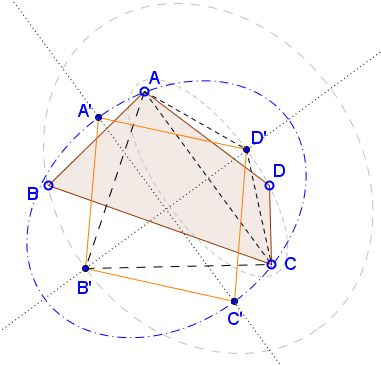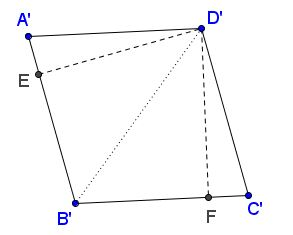# Isoperimetric Theorem for Quadrilaterals II

### Introduction

Among all quadrilaterals with the same perimeter, square has the largest area.

or, equivalently,

Among all quadrilaterals with the same area, square has the smallest perimeter.

If $P$ is the perimeter and $A$ area of a $2D$ figure, then, for square, $P^{2}=16A.$ The above statement, known as isoperimetric theorem is equivalent to the inequality, $P^{2}\ge 16A,$ for any quadrilateral, with equality only for square.

For a non-convex quadrilateral, one of the diagonals passes in its exterior. If, say, $AC$ is such a diagonal, then quadrilateral $ABCD',$ where $D'$ is the mirror image of $D$ in $AC,$ has the same perimeter as $ABCD$ but larger area, obviously.

For convex quadrilateral, the proof proceeds in three steps. Starting with an arbitrary quadrilateral, which is not a kite, we'll find a kite with the same perimeter but larger area. For a kite which is not a rhombus, we'll find a rhombus with the same perimeter but larger area. Finally, for a rhombus which is not a square, we'll find a square with the same perimeter but larger area.

I shall use $[X]$ to denote the area of shape $X.$

If, for example, $AD\ne CD,$ draw an ellipse with foci at $A$ and $C$ passing through $D$. Let the perpendicular bisector of $AC$ cross the ellipse in $D'$ on the same side of the axis $AC$ as $D.$ Then $AD+CD=AD'+CD'$ but, in addition, $[ACD']\gt [ACD]$ because the two triangles have the same base $AC$ with the altitude of one longer than that of the other.In case $AB=BC$ we may proceed to the next step. If not, as before, we draw an ellipse with foci at $A$ and $C$ passing through $B$ and find $B'$ such that $AB+BC=AB'+B'C$ and $[ACB']\gt [ACB].$

Putting the triangles together we see that $AB'CD'$ has the same perimeter as $ABCD$ but larger area.

### Kite to rhombus

We start with quadrilateral $AB'CD'$ in which $AB'=B'C$ and $AD'=CD'.$ If, say, $AB'=AD'$ the quadrilateral is a rhombus and we may proceed to the next step. If not, draw an ellipse with foci at $B'$ and $D'$ and passing through $A$. By symmetry, $C$ will also lie on th ellipse.As on the previous step me may choose $A'$ (and simultaneously $C'$) such that $A'B'C'D'$ has the same perimeter as $AB'CD'$ but larger area while having all four sides equal.

### Rhombus to square

Given rhombus $A'B'C'D',$ consider the areas of two triangles $A'B'D'$ and $B'C'D'.$ Their areas are half the product of their bases $A'B'$ and $B'C'$ by their respective altitudes $D'E$ and $D'F.$ Both altitudes do not exceed the sides $A'D'$ and $C'D'$ of the triangles. They are equal to the side only when $A'B'C'D'$ is a square.### Acknowledgment

The proof is due to A. M. Fink (The Mathematical Gazette, v 98, n 543 Nov 2014, 504) from Iowa State University.

An alternative proof proceeds through the sequence

$quadrilateral\rightarrow parallelogram\rightarrow rectangle\rightarrow square.$

• Isoperimetric Theorem and Inequality
• An Isoperimetric theorem
• Isoperimetric theorem and its variants
• Isoperimetric Property of Equilateral Triangles
• Isoperimetric Property of Equilateral Triangles II
• Maximum Area Property of Equilateral Triangles
•